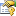﻿ SupportVectorReductionBase(TModel, TKernel, TInput) ClassExact support vector reduction through linear dependency elimination.Inheritance Hierarchy
SystemObject
Accord.MachineLearning.VectorMachines.LearningBaseSupportVectorCalibrationTModel, TKernel, TInput
Accord.MachineLearning.VectorMachines.LearningSupportVectorReductionBaseTModel, TKernel, TInput
Accord.MachineLearning.VectorMachines.LearningSupportVectorReduction
Accord.MachineLearning.VectorMachines.LearningSupportVectorReductionTKernel
Accord.MachineLearning.VectorMachines.LearningSupportVectorReductionTKernel, TInput

Namespace:  Accord.MachineLearning.VectorMachines.Learning
Assembly:  Accord.MachineLearning (in Accord.MachineLearning.dll) Version: 3.8.0Syntax
```public class SupportVectorReductionBase<TModel, TKernel, TInput> : BaseSupportVectorCalibration<TModel, TKernel, TInput>
where TModel : SupportVectorMachine<TKernel, TInput>
where TKernel : Object, IKernel<TInput>
where TInput : ICloneable
```

#### Type Parameters

TModel
TKernel
TInput

The SupportVectorReductionBaseTModel, TKernel, TInput type exposes the following members.Constructors
NameDescriptionSupportVectorReductionBaseTModel, TKernel, TInput(TModel)
Creates a new SupportVectorReduction algorithm.SupportVectorReductionBaseTModel, TKernel, TInput(ISupportVectorMachineTInput)
Creates a new SupportVectorReduction algorithm.
TopProperties
NameDescriptionInput
Gets or sets the input vectors for training.
(Inherited from BaseSupportVectorCalibrationTModel, TKernel, TInput.)IsLinear
Gets whether the machine being learned is linear.
(Inherited from BaseSupportVectorCalibrationTModel, TKernel, TInput.)Kernel
Gets the machine's IKernel function.
(Inherited from BaseSupportVectorCalibrationTModel, TKernel, TInput.)Model
Gets the machine to be taught.
(Inherited from BaseSupportVectorCalibrationTModel, TKernel, TInput.)Output
Gets or sets the output labels for each training vector.
(Inherited from BaseSupportVectorCalibrationTModel, TKernel, TInput.)Threshold
Gets or sets the minimum threshold that is used to determine whether a weight will be kept in the machine or not. Default is 1e-12.Token
Gets or sets a cancellation token that can be used to stop the learning algorithm while it is running.
(Inherited from BaseSupportVectorCalibrationTModel, TKernel, TInput.)
TopMethods
NameDescriptionCreate
Creates an instance of the model to be learned. Inheritors of this abstract class must define this method so new models can be created from the training data.
(Inherited from BaseSupportVectorCalibrationTModel, TKernel, TInput.)Equals
Determines whether the specified object is equal to the current object.
(Inherited from Object.)Finalize
Allows an object to try to free resources and perform other cleanup operations before it is reclaimed by garbage collection.
(Inherited from Object.)GetHashCode
Serves as the default hash function.
(Inherited from Object.)GetType
Gets the Type of the current instance.
(Inherited from Object.)InnerRun
Runs the learning algorithm.
(Overrides BaseSupportVectorCalibrationTModel, TKernel, TInputInnerRun.)Learn
Learns a model that can map the given inputs to the given outputs.Learn(TInput, Boolean, Double)
Learns a model that can map the given inputs to the given outputs.
(Inherited from BaseSupportVectorCalibrationTModel, TKernel, TInput.)Learn(TInput, Boolean, Double)
Learns a model that can map the given inputs to the given outputs.
(Inherited from BaseSupportVectorCalibrationTModel, TKernel, TInput.)Learn(TInput, Double, Double)
Learns a model that can map the given inputs to the given outputs.
(Inherited from BaseSupportVectorCalibrationTModel, TKernel, TInput.)Learn(TInput, Int32, Double)
Learns a model that can map the given inputs to the given outputs.
(Inherited from BaseSupportVectorCalibrationTModel, TKernel, TInput.)Learn(TInput, Int32, Double)
Learns a model that can map the given inputs to the given outputs.
(Inherited from BaseSupportVectorCalibrationTModel, TKernel, TInput.)MemberwiseClone
Creates a shallow copy of the current Object.
(Inherited from Object.)Run Obsolete.
Obsolete.
(Overrides BaseSupportVectorCalibrationTModel, TKernel, TInputRun.)ToString
Returns a string that represents the current object.
(Inherited from Object.)
TopExtension Methods
NameDescriptionHasMethod
Checks whether an object implements a method with the given name.
(Defined by ExtensionMethods.)IsEqual
Compares two objects for equality, performing an elementwise comparison if the elements are vectors or matrices.
(Defined by Matrix.)To(Type)Overloaded.
Converts an object into another type, irrespective of whether the conversion can be done at compile time or not. This can be used to convert generic types to numeric types during runtime.
(Defined by ExtensionMethods.)ToTOverloaded.
Converts an object into another type, irrespective of whether the conversion can be done at compile time or not. This can be used to convert generic types to numeric types during runtime.
(Defined by ExtensionMethods.)
TopExamples

The following example shows how to reduce the number of support vectors in a SVM by removing vectors which are linearly dependent between themselves.

```// Example AND problem
double[][] inputs =
{
new double[] { 0, 0 }, // 0 and 0: 0 (label -1)
new double[] { 0, 1 }, // 0 and 1: 0 (label -1)
new double[] { 1, 0 }, // 1 and 0: 0 (label -1)
new double[] { 1, 1 }  // 1 and 1: 1 (label +1)
};

// Dichotomy SVM outputs should be given as [-1;+1]
int[] labels =
{
// 0,  0,  0, 1
-1, -1, -1, 1
};

// Instantiate a new learning algorithm for SVMs
var smo = new SequentialMinimalOptimization<Linear>()
{

// Set up the learning algorithm
Complexity = 100.0
};

// Run
var svm = smo.Learn(inputs, labels);

int numberBefore = svm.SupportVectors.Length; // should be

double errorBefore = new ZeroOneLoss(labels).Loss(svm.Decide(inputs)); // should be 0

// At this point we have the weighted support vectors
//     w        sv        b
//   (+4)  *  (1,1)      -3
//   (-2)  *  (1,0)
//   (-2)  *  (0,1)
//
// However, it can be seen that the last SV can be written
// as a linear combination of the two first vectors:
//
//   (0,1) = (1,1) - (1,0)
//
// Since we have a linear space (we are using a linear kernel)
// this vector could be removed from the support vector set.
//
// f(x) = sum(alpha_i * x * x_i) + b
//      = 4*(1,1)*x - 2*(1,0)*x - 2*(0,1)*x             - 3
//      = 4*(1,1)*x - 2*(1,0)*x - 2*((1,1) - (1,0))*x   - 3
//      = 4*(1,1)*x - 2*(1,0)*x - 2*(1,1)*x + 2*(1,0)*x - 3
//      = 4*(1,1)*x - 2*(1,0)*x - 2*(1,1)*x + 2*(1,0)*x - 3
//      = 2*(1,1)*x - 3
//      = 2*x1 + 2*x2 - 3
//

// Clone the original machine so we don't change it
var clone = (SupportVectorMachine<Linear>)svm.Clone();

// Create a support vector reduction algorithm
var svr = new SupportVectorReduction<Linear>(clone);

// Reduce the number of support vectors
var svm2 = svr.Learn();

int numberAfter = svm2.SupportVectors.Length; // should be 1

// Compute the new error
double errorAfter = new ZeroOneLoss(labels).Loss(svm2.Decide(inputs)); // should still be 0```See Also